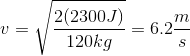# AP Physics C: Mechanics : Kinetic Energy

## Example Questions

### Example Question #1 : Kinetic Energy

A train car with a mass of 2400 kg starts from rest at the top of a 150 meter-high hill. What will its velocity be when it reaches the bottom of the hill, assuming that the bottom of the hill is the reference level.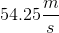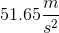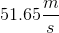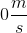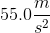Explanation:

The law of conservation of energy states: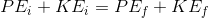If the car starts at rest, then the initial kinetic energy = 0 J.

If the car ends at the reference height, the final potential energy = 0 J.

Subsituting these values, the equation becomes: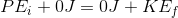The initial potential energy can be determined by: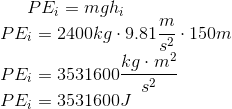The final kinetic energy equation is: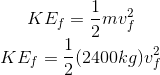Substituting the initial potential energy and final kinetic energy into our modified conservation of energy equation, we get: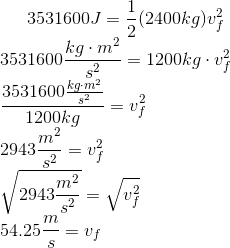### Example Question #1 : Energy

An object has a mass of 5kg and has a position described by the given function: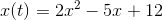What is the object's kinetic energy after two seconds?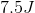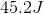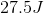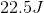Explanation:

Kinetic energy is defined by the equation: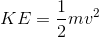Taking the derivative of the position function allows us to obtain the velocity function: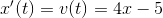We can now determine the velocity after two seconds: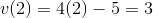Now that we know our velocity, we can solve for the kinetic energy.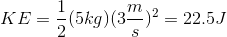### Example Question #3 : Energy

An object starts from rest and accelerates at a rate of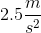. If the object has a mass of 10kg, what is its kinetic energy after three seconds?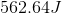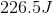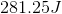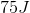Explanation:

Kinetic energy is given by the equation:We can find the velocity using the given acceleration and time: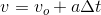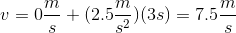Use this velocity to find the kinetic energy after three seconds: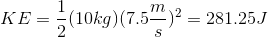### Example Question #4 : Energy

A 120kg box has a kinetic energy of 2300J. What is its velocity?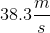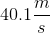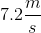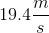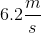Explanation:

The formula for kinetic energy of an object is:The problem gives us the mass and the kinetic energy, and asks for the velocity, so we can rearrange the equation: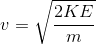Use our given values for kinetic energy and mass to solve: